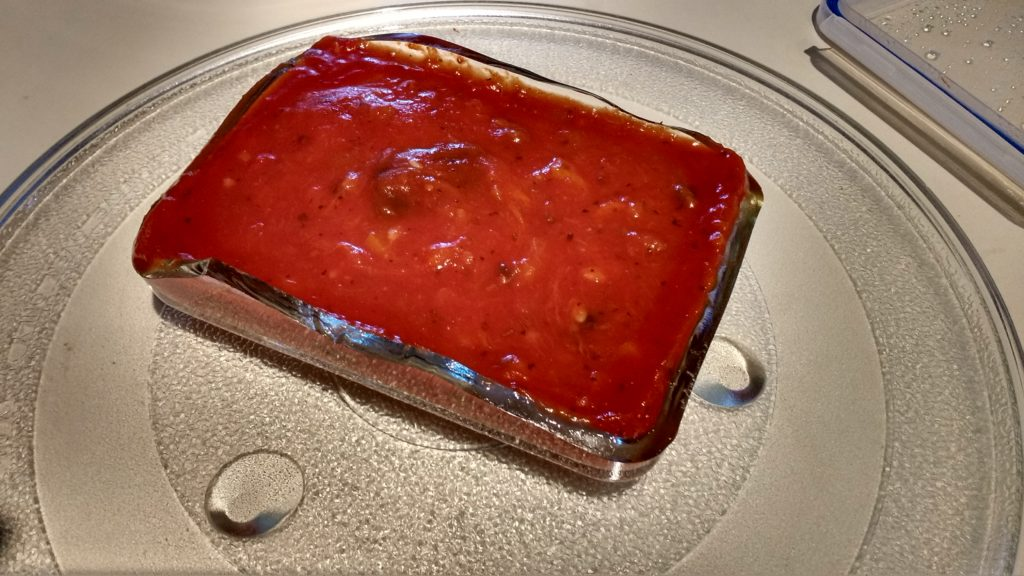## An interesting heat fracture

November 5, 2018 Incoherent ramblings , ,

Check out this break after 2 minutes in the microwave:I’d nuked it with the lid on, but askew, so that the sauce wouldn’t splash, but heat could still get out. Look at how close to the sauce line the break occurred:I was actually able to take off the top half of the container, and nothing spilled!  It was such a clean break that I probably could have eaten the sauce in the center of the container (I didn’t, and threw it all out to be safe).

I’d guess there was a hairline fracture somewhere around the “sauce line” and the heat differential with this particular loading was enough to stress that defect enough to break all the way.

## PHY2403 (QFT I). Pondering the ground state bra formula.

November 5, 2018 phy2403 , ,

In lecture 14 we found

\begin{equation}\label{eqn:qftLecture15:60}
\ket{\Omega}
=
\evalbar{
\frac{ U(t_0, -T) \ket{0} }
{
e^{-i E_0(T – t_0)} \braket{\Omega}{0}
}
}{T \rightarrow \infty(1 – i \epsilon)},
\end{equation}
and it was stated that we can also show that
\begin{equation}\label{eqn:qftLecture15:80}
\bra{\Omega}
=
\evalbar{
\frac{ \bra{0} U(T, t_0) }
{
e^{-i E_0(T – t_0)} \braket{0}{\Omega}
}
}{T \rightarrow \infty(1 – i \epsilon)}.
\end{equation}

This second statement is actually not obvious since
\begin{equation}\label{eqn:braOmega:100}
\lr{
\frac{ U(t_0, -T) \ket{0} }
{
e^{-i E_0(T – t_0)} \braket{\Omega}{0}
}
}^\dagger
=
\frac{ \bra{0} U(-T, t_0) \ket{0} }
{
e^{+i E_0(T – t_0)} \braket{0}{\Omega}
}
\end{equation}

My first thought was that I’d written down \ref{eqn:qftLecture15:80} in my notes wrong, but this is actually also consistent with , which our Prof is following loosely (i.e. he is explicitly filling in many of the holes in that dense little 800 page book).

The resolution of this inconsistency is that the limit point $$\infty(1 – i \epsilon)$$ doesn’t work if you just conjugate, and you’d also have to conjugate that limit, so while
\ref{eqn:braOmega:100} is correct, it is only part of the story, and should really be stated as
\begin{equation}\label{eqn:braOmega:120}
\bra{\Omega}
=
\evalbar{
\frac{ \bra{0} U(-T, t_0) \ket{0} }
{
e^{+i E_0(T – t_0)} \braket{0}{\Omega}
}
}{T \rightarrow \infty(1 + i \epsilon)}.
\end{equation}
This is awkward because now our expressions for $$\bra{\Omega}$$ and $$\ket{\Omega}$$ approach $$T$$ from different directions, and we want to evaluate both with a single limiting argument.

To resolve this, we really have to start back with the identity expansion we used in lecture 14
\begin{equation}\label{eqn:braOmega:140}
\begin{aligned}
\bra{0} e^{-i H T}
&=
\lr{
\braket{0}{\Omega}\bra{\Omega}
+ {\int\kern-1em\sum}_n \braket{0}{n} \bra{n}
}
e^{-i H T} \\
&=
\braket{0}{\Omega}\bra{\Omega}
e^{-i E_0 T}
+ {\int\kern-1em\sum}_n \braket{0}{n} \bra{n} e^{-i E_n T}.
\end{aligned}
\end{equation}
We argued (as does the text) that approaching to as $$T( 1 – i \epsilon)$$ kills off the energetic states since
\begin{equation}\label{eqn:braOmega:160}
\bra{n} e^{-i E_n T}
\rightarrow
\bra{n} e^{-i E_n T} e^{-E_n T \epsilon}
\end{equation}
and the exponential damping factor is smaller for each $$E_n > E_0$$, so it can be neglected in the large $$T$$ limit, leaving
\begin{equation}\label{eqn:braOmega:180}
\bra{0} e^{-i H T}
=
\lim_{T \rightarrow \infty(1 – i \epsilon)}
\braket{0}{\Omega}\bra{\Omega}.
\end{equation}
As we did for $$\ket{\Omega}$$ we can shift the large time $$T$$ by a small constant (this time $$-t_0$$ instead of $$t_0$$), to give
\begin{equation}\label{eqn:braOmega:200}
\begin{aligned}
\bra{\Omega}
&=
\lim_{T \rightarrow \infty(1 – i \epsilon)}
\frac{ \bra{0} e^{-i H T} }
{
\braket{0}{\Omega} e^{-i E_0 T}
} \\
&\approx
\lim_{T \rightarrow \infty(1 – i \epsilon)}
\frac{ \bra{0} e^{-i H (T – t_0)} }
{
\braket{0}{\Omega} e^{-i E_0 (T – t_0)}
} \\
&=
\lim_{T \rightarrow \infty(1 – i \epsilon)}
\frac{ \bra{0} e^{i H_0( T – t_0)} e^{-i H (T – t_0)} }
{
\braket{0}{\Omega} e^{-i E_0 (T – t_0)}
} \\
&=
\lim_{T \rightarrow \infty(1 – i \epsilon)}
\frac{ \bra{0} U(T, t_0) }
{
\braket{0}{\Omega} e^{-i E_0 (T – t_0)}
},
\end{aligned}
\end{equation}
where the projective property $$\bra{0} e^{i H_0 \alpha} = \bra{0}$$ has been used to insert a no-op (i.e. $$\bra{0} H_0 = 0$$). This recovers the result stated in class (also: eq. (4.29) in the text.)

# References

 Michael E Peskin and Daniel V Schroeder. An introduction to Quantum Field Theory. Westview, 1995.